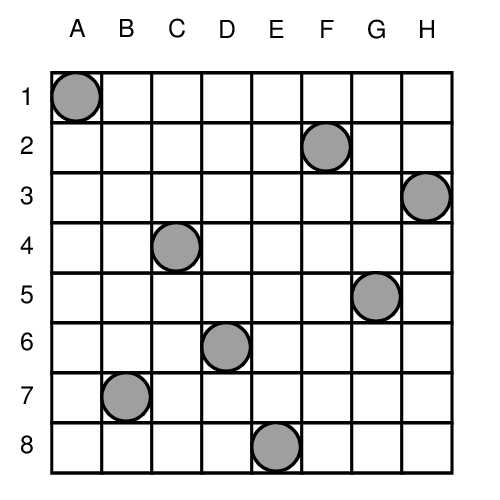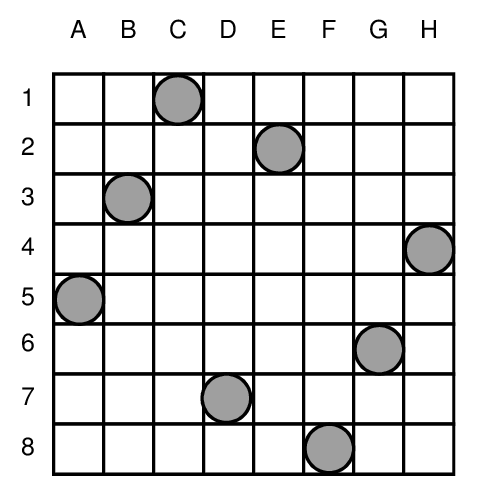# Define a New Constraint using Demons¶

Demons are useful to define new constraints.

During constraint propagation, some events (i.e. changes in CSint variables domains) will occur on CSint variables. These events are categorized into four exclusive types:

Known:

the domain has been reduced to one value (the CSint variable is instantiated);

Ex.: cs_EQ(vint, 0); /* raise a Known evnent */

NewMin:

the minimum value of the domain has been changed (became strictly greater);

Ex.: cs_GT(vint, 0);

NewMax:

the maximum value of the domain has been changed (became strictly lower);

Ex: cs_LT(vint, 0);

Neq:

one value has been removed from the domain;

Ex: cs_NEQ(vint, 0);

These events are exclusive, that is:

• a known event will not raise neither a newMin, newMax, nor a neq event;
• newMin nor newMax events will not raise any neq event;

For example,

CSint *vint = cs_createCSint(0, 10);
cs_GE(vint, 10);


will raise a known event (vint will be instantiated to 10) but not a newMin event.

CSint *vint = cs_createCSint(0, 10);
cs_NEQ(vint, 0);


will raise a newMin event (vint will be strictly greater than 1), but not a neq event.

For example, here is the code of the well-known N-Queens problem, implemented using cs_eventKnown().

(The 8-Queens problem consists in positioning 8 queens on a chess board in such a way that no queen is on the same horizontal, vertical or diagonal line as another.)

When a queen is positioned (i.e. when a CSint variable allvars[i] is known), then we have to express that no other queen should be on the same row nor diagonal.

#include <stdlib.h>
#include "iz.h"

// This function is called when allvars[index] is instantiated
// It returns FALSE if Constraints Propagation fails
IZBOOL knownQueen(int val, int index, CSint **allvars, int NbQueens, void *extra)
{
int i;

for (i = 0; i < NbQueens; i++) {
if (i != index) {
CSint *var = allvars[i];

if (!cs_NEQ(var, val)) return FALSE;  // No queens on the same row
if (!cs_NEQ(var, val + index - i)) return FALSE; //No queens on the same diagonal
if (!cs_NEQ(var, val + i - index)) return FALSE;
}
}
return TRUE;
}

int main(int argc, char **argv)
{
int NbQueens = (argc > 1) ? atoi(argv) : 8;
CSint **allvars;

cs_init();

allvars = cs_createCSintArray(NbQueens, 1, NbQueens);

cs_eventKnown(allvars, NbQueens, knownQueen, NULL);
cs_search(allvars, NbQueens, cs_findFreeVarNbElementsMin);
cs_printf("%A\n", allvars, NbQueens);

cs_printStats();

cs_end();

return 0;
}


The corresponding output is:

1, 7, 4, 6, 8, 2, 5, 3

Nb Fails = 11
Nb Choice Points = 20
Heap Size = 1024


Solution “1, 7, 4, 6, 8, 2, 5, 3” is visualized as following:The 8-Queens problem has 92 solutions, which can be found using

cs_findAll(allvars, NbQueens, cs_findFreeVarNbElementsMin, found)


instead of

cs_search(allvars, NbQueens, cs_findFreeVarNbElementsMin);


found() being defined as:

void found(CSint **allvars, int NbQueens)
{
static NbSolutions = 0;

printf("Solution %d\n", ++NbSolutions);
cs_printf("%A\n\n", allvars, NbQueens);
}


The 92 solutions are found within 289 backtracks. We can notice the 55th one, which is interesting: 5, 3, 1, 7, 2, 8, 6, 4All the cs_event() functions take as their third argument the reference to the function that will called when the event will be triggered. These functions (allKnown(), known(), newMin(), newMax(), neq()) must return TRUE or FALSE.